# 使用技術

1. 物件偵測（ Object detection）：

1. 物件追蹤 （Object tracking）

1. 影像處理

# 車輛偵測定位及分類

## 車輛追蹤及計算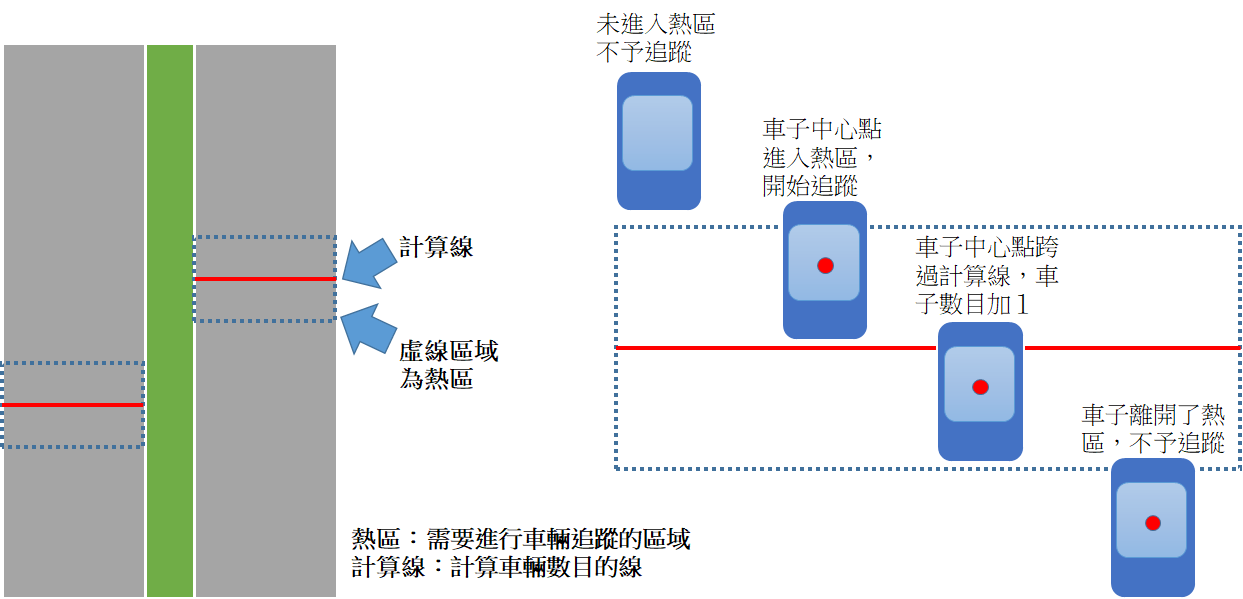• 判斷此車輛的位置是否在熱區的範圍。
• 若處於熱區範圍，則與上一個frame的車輛進行比對，計算該車中心點與上一個frame所有車輛的中心點距離最短是那一台。
• 若非處於熱區範圍則略過不處理。
• 由於我們的熱區是定義於道路中間位置，因此理論上該熱區不會有突然新出現的車輛，每一台車應該能找到其一個frame的所在位置。
• 經由上下frame得到該車的行徑方向，並將該方向的車輛數目加1。
• 車子行徑的方向，可由上下frame車子的中心點(X,Y)的變化來判斷：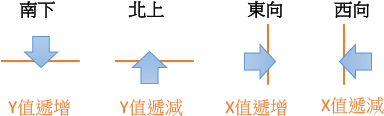# 實例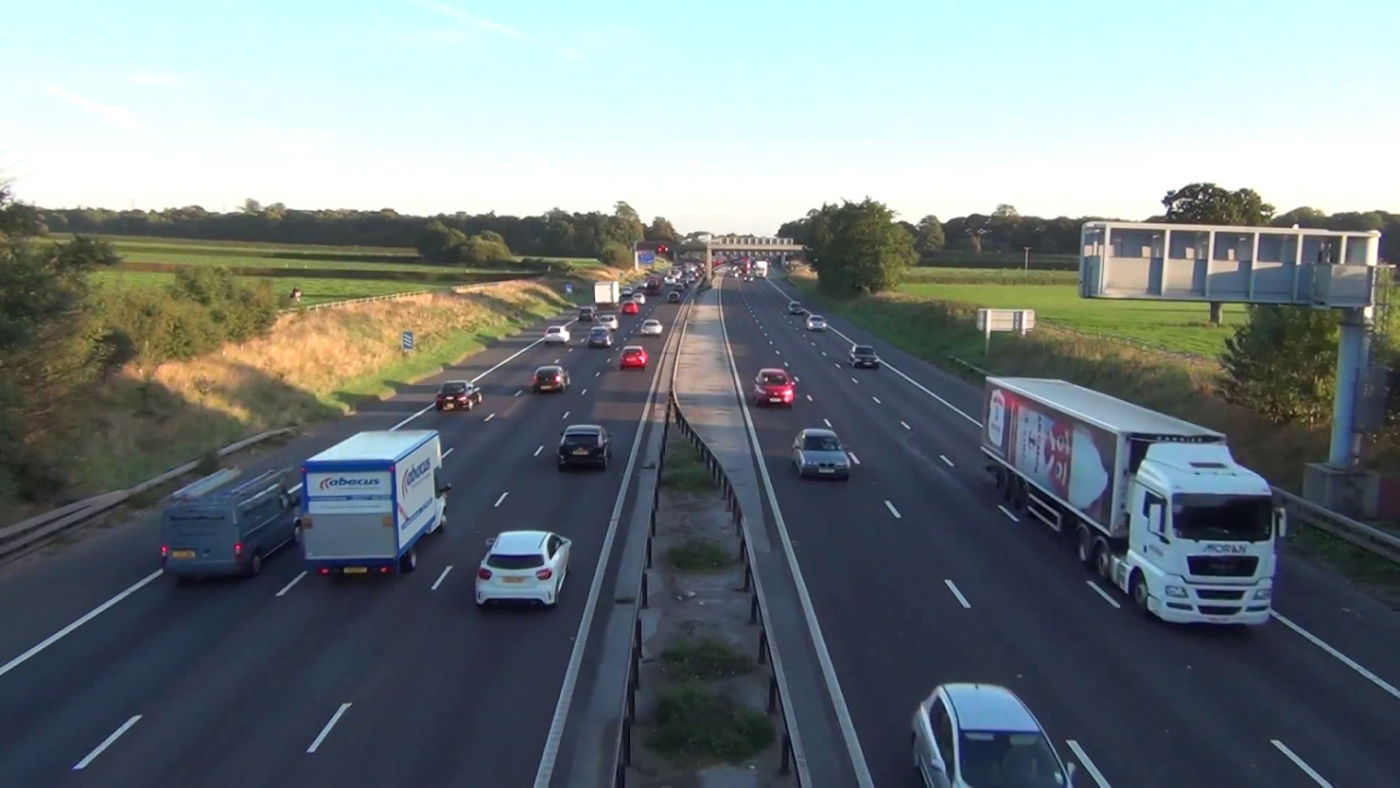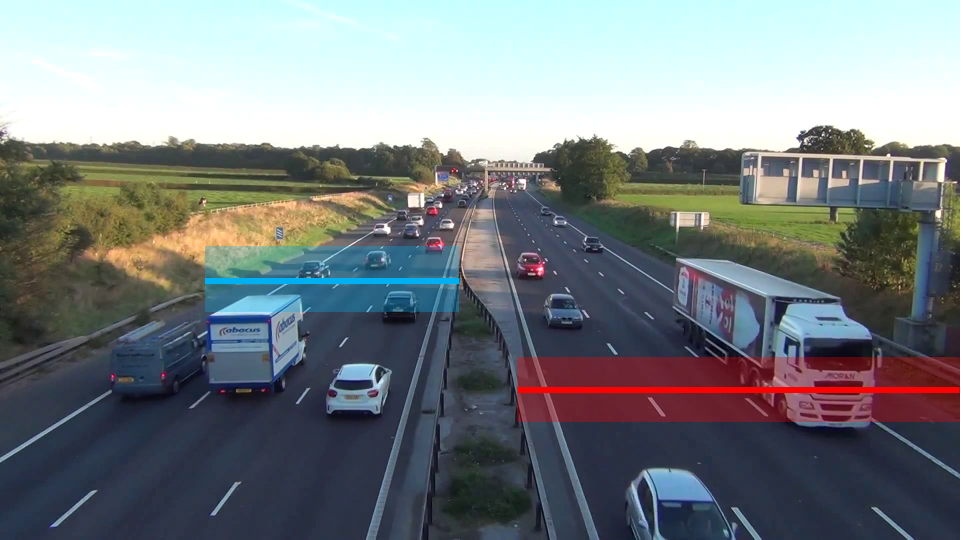``def in_range_N2S(bbox):    #only calculate the cars (from south to north) run across the line and not over Y +- calculateRange_y    x = bbox    y = bbox    w = bbox    h = bbox    cx = int(x+(w/2))    cy = int(y+(h/2))    if(cx>=calculateLine2 and cx<=calculateLine2 and \        cy>calculateLine2-calculateRange_y and cy<=(calculateLine2+calculateRange_y) ):        return True    else:        return Falseif(in_range_S2N(box)==True or in_range_N2S(box)==True):    now_IDs.append(id)    now_BBOXES.append(box)    now_CENTROIDS.append(bbox2Centroid(box))    now_LABELS.append(labelName)    now_COUNTED.append(False)    cv2.rectangle(frame, (box, box), (box+box, box+box), (0,255,0), 2)``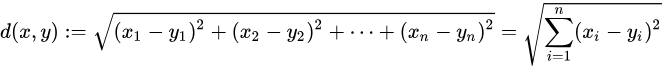``def count_Object(centroid_last, centroid_now):    distances = []    for cent_last in centroid_last:        smallist = 99999.0        smallist_id = 0        dist = 0.0                for id, cent_now in enumerate(centroid_now):            dist = distance(cent_now, cent_last)            if(dist<=smallist):                smallist = dist                smallist_id = id        distances.append(smallist_id)    return distances``

``for id, now_id in enumerate(obj_target):    #if last Y is under the line and now Y is above or on the line, then count += 1    print(last_CENTROIDS[id], calculateLine2, now_CENTROIDS[now_id], calculateLine2)    UP = last_CENTROIDS[id]>calculateLine1 and now_CENTROIDS[now_id]<=calculateLine1    DOWN = last_CENTROIDS[id]<calculateLine2 and now_CENTROIDS[now_id]>=calculateLine2    if( UP is True):        if(now_LABELS[now_id]=="truck"):            count_Truck1 += 1        elif(now_LABELS[now_id]=="car"):            count_Car1 += 1        elif(now_LABELS[now_id]=="bus"):            count_Bus1 += 1        elif( DOWN is True):            if(now_LABELS[now_id]=="truck"):                count_Truck2 += 1            elif(now_LABELS[now_id]=="car"):                count_Car2 += 1            elif(now_LABELS[now_id]=="bus"):                count_Bus2 += 1``

# 非垂直或水平的道路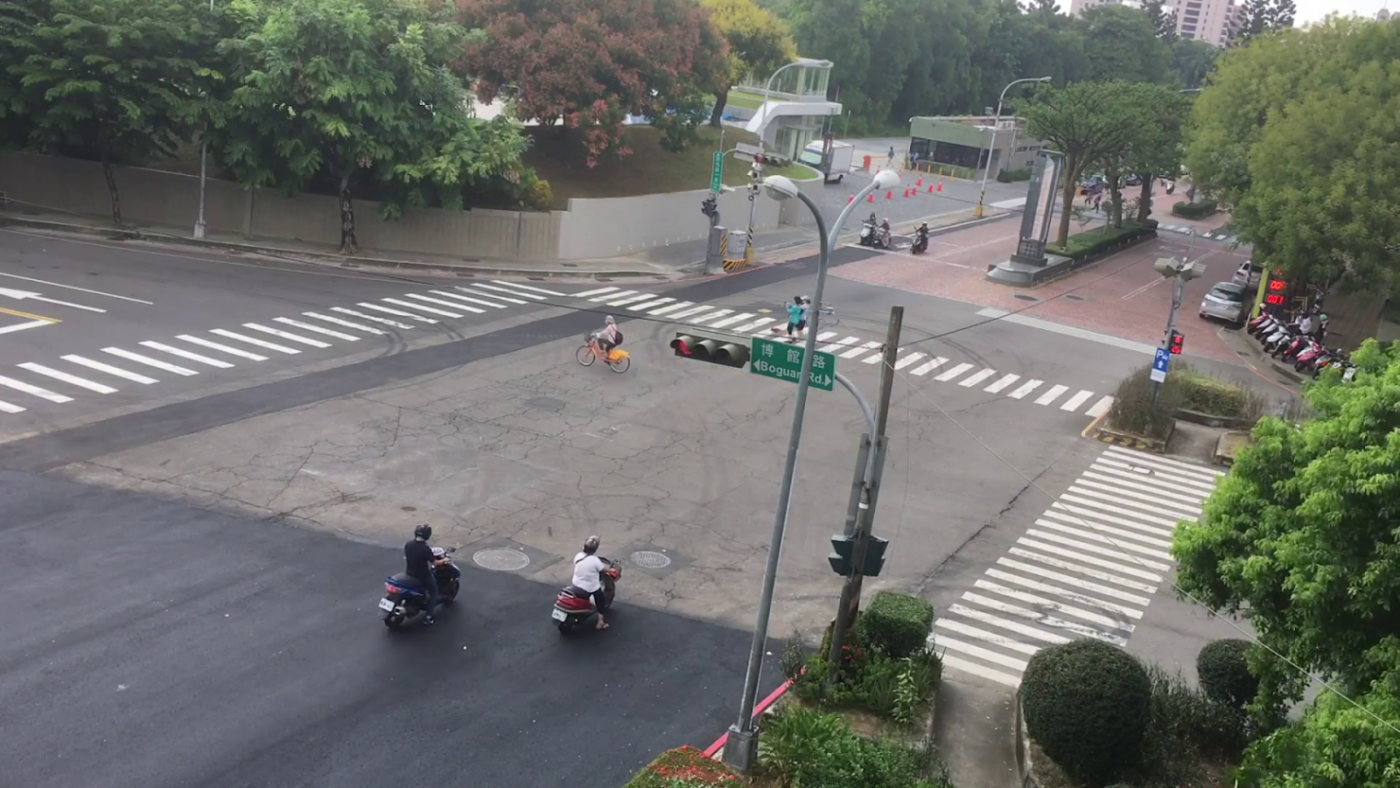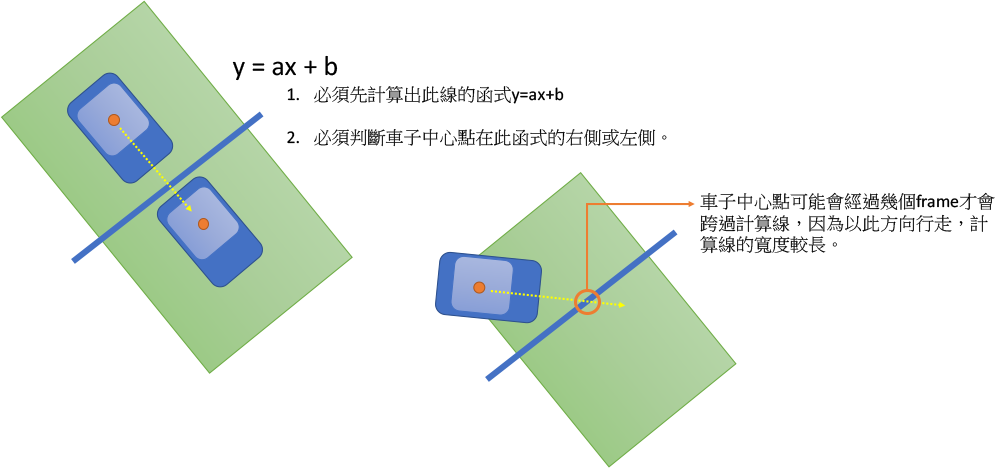# 以隱藏的色線來取代計算線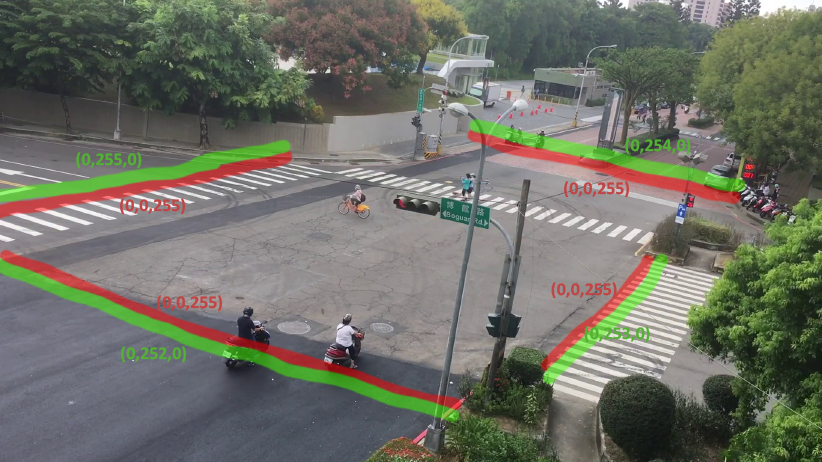1. 紅色與綠色的交界處就類似我們之前的計算線，其不同色系的交界處永遠為1，不會有寬度不均的問題。
2. 取得車輛中心點在隱藏層的顏色，比起推論線性函式及計算該點位於該線的何種方位來得簡單且直覺多了。
3. 提供自行定義的界面，讓使用者可在相片中抹上顏色或劃線，自行加入不規則形狀的禁區或熱區。例如，我們可以在如下的草坪區加入隱藏的色區，用於偵測是否有人侵入。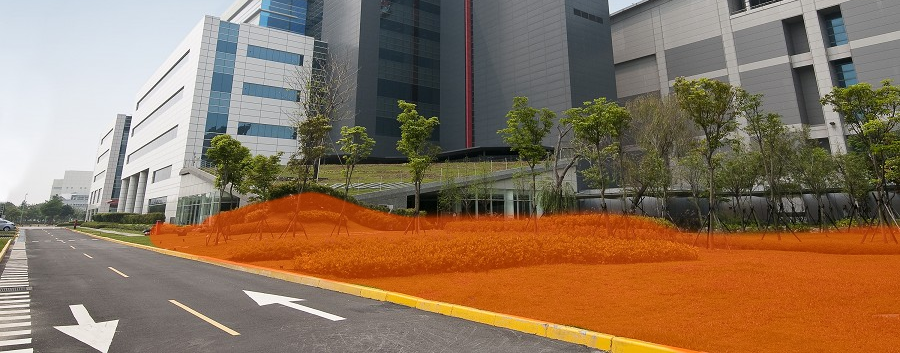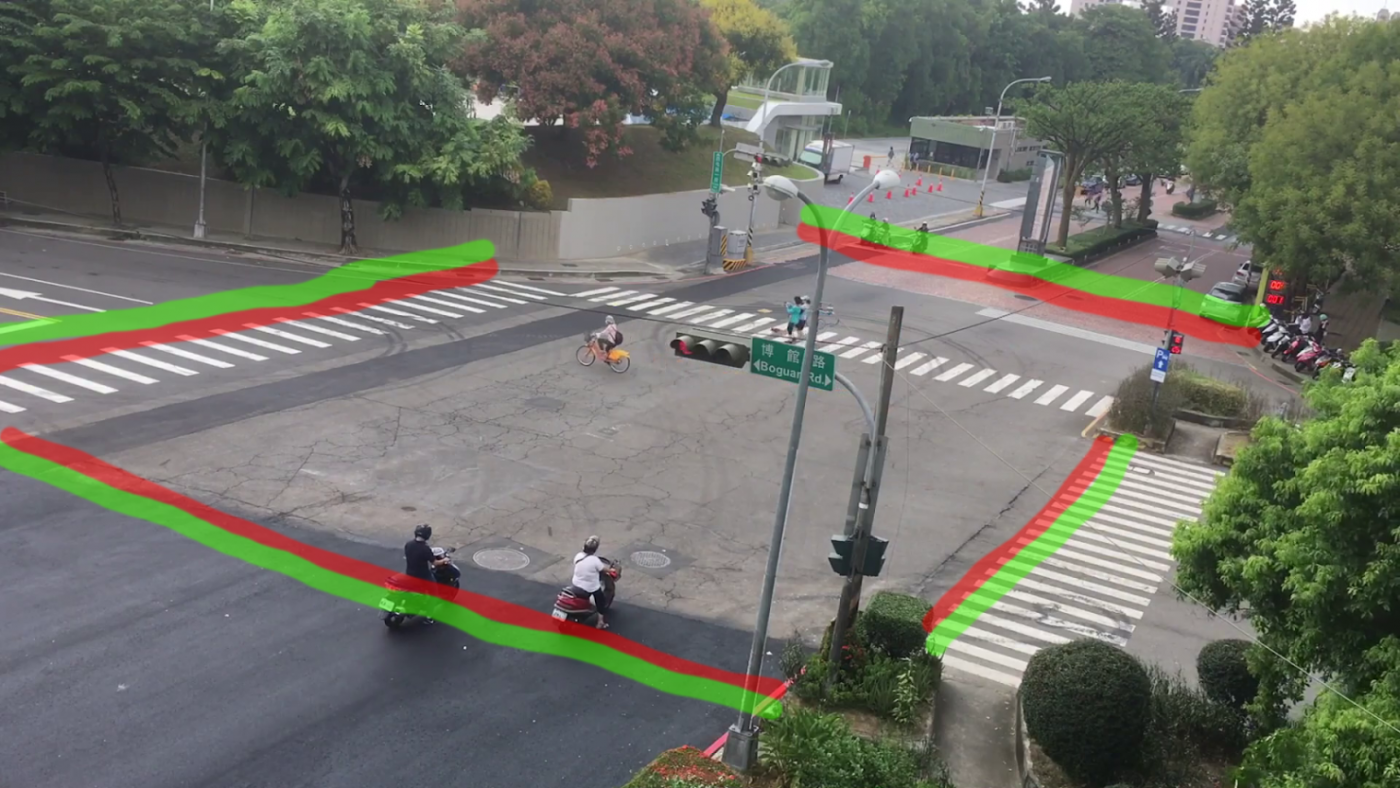``calculateLine1 = [(36, 610), (1126, 900)]  #[(from(x,y), to(x,y)]calculateLine2 = [(820, 350), (1574, 530)]  #[(from(x,y), to(x,y)]calculateLine3 = [(830, 380), (0, 580)]  #[(from(x,y), to(x,y)]calculateLine4 = [(1532, 590), (1224, 880)]  #[(from(x,y), to(x,y))def draw_CalculateLine(frame):    cv2.line(frame, (calculateLine1,calculateLine1+20), (calculateLine1,calculateLine1+25), (0, 255, 0), 40)    cv2.line(frame, (calculateLine2,calculateLine2-20), (calculateLine2,calculateLine2-25), (0, 255, 0), 40)    cv2.line(frame, (calculateLine3,calculateLine3-20), (calculateLine3,calculateLine3-25), (0, 255, 0), 40)    cv2.line(frame, (calculateLine4,calculateLine4+25), (calculateLine4,calculateLine4+35), (0, 255, 0), 45)    cv2.line(frame, (calculateLine1,calculateLine1), (calculateLine1,calculateLine1), (0, 0, 255), 40)    cv2.line(frame, (calculateLine2,calculateLine2), (calculateLine2,calculateLine2), (0, 0, 254), 40)    cv2.line(frame, (calculateLine3,calculateLine3), (calculateLine3,calculateLine3), (0, 0, 253), 40)    cv2.line(frame, (calculateLine4,calculateLine4), (calculateLine4,calculateLine4), (0, 0, 252), 40)    return frameframeLayout = frame.copy()frameLayout = draw_CalculateLine(frameLayout)``

``color_now = frameLayout[now_CENTROIDS[now_id],now_CENTROIDS[now_id]]color_last = frameLayout[last_CENTROIDS[id],last_CENTROIDS[id]]UP_1 = (color_now == [0,0,255]).all() and (color_last == [0,255,0]).all()DOWN_1 = (color_last == [0,0,255]).all() and (color_now==[0,255,0]).all()UP_2 = (color_now == [0,0,254]).all() and (color_last == [0,255,0]).all()DOWN_2 = (color_last == [0,0,254]).all() and (color_now==[0,255,0]).all()UP_3 = (color_now == [0,0,253]).all() and (color_last == [0,255,0]).all()DOWN_3 = (color_last == [0,0,253]).all() and (color_now==[0,255,0]).all()UP_4 = (color_now == [0,0,252]).all() and (color_last == [0,255,0]).all()DOWN_4 = (color_last == [0,0,252]).all() and (color_now==[0,255,0]).all()``